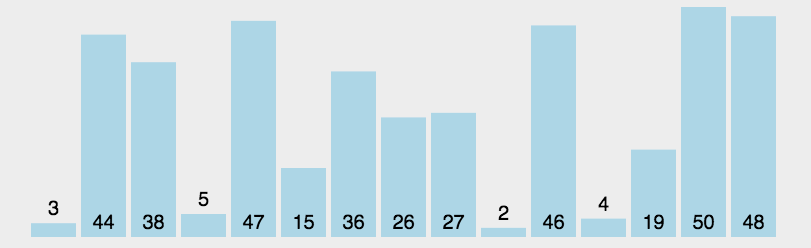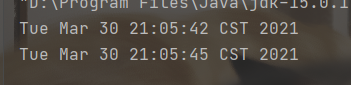• JAVA实现选择排序 1、选择排序概念 选择排序，在一组无序数组中挑选当前最小的数字然后放到第一个。后续的数组依次按照该方式进行排序。整个排序需要N-1次！该方式可以类比小时候进行的体育队伍。长的矮的同学往前面...
JAVA实现选择排序
1、选择排序概念
选择排序，在一组无序数组中挑选当前最小的数字然后放到第一个。后续的数组依次按照该方式进行排序。整个排序需要N-1次！该方式可以类比小时候进行的体育队伍。长的矮的同学往前面站！

2、图解选择排序3、选择排序的时间复杂度
4、选择排序的代码实现


展开全文• java实现选择排序 选择排序（Selection sort）是一种简单直观的排序算法。 工作原理： 第一次从待排序的数据元素中选出最小（或最大）的一个元素，存放在序列的起始位置，然后再从剩余的未排序元素中寻找到最小（大...
java实现选择排序

选择排序（Selection sort）是一种简单直观的排序算法。
工作原理： 第一次从待排序的数据元素中选出最小（或最大）的一个元素，存放在序列的起始位置，然后再从剩余的未排序元素中寻找到最小（大）元素，然后放到已排序的序列的末尾。以此类推，直到全部待排序的数据元素的个数为零。选择排序是不稳定的排序方法。

排序思路：
首先在未排序序列中找到最小（大）元素，存放到排序序列的起始位置，然后，再从剩余未排序元素中继续寻找最小（大）元素，然后放到已排序序列的末尾。以此类推，直到所有元素均排序完毕。

时间复杂度：

选择排序的交换操作介于 0 和 (n - 1)次之间。选择排序的比较操作为 n (n - 1） / 2 次之间。选择排序的赋值操作介于 0 和 3 (n - 1）次之间。比较次数O(n^2），比较次数与关键字的初始状态无关，总的比较次数N=(n-1）+(n-2）+…+1=n*(n-1）/2。交换次数O(n），最好情况是，已经有序，交换0次；最坏情况交换n-1次，逆序交换n/2次。交换次数比冒泡排序少多了，由于交换所需CPU时间比比较所需的CPU时间多，n值较小时，选择排序比冒泡排序快。

java代码实现：
//选择排序  从小到大排
public class SelectSort {
public static void main(String[] args) {
int[] arr = new int;
for (int i = 0; i < 80000; i++) {
int random = (int) (Math.random() * 100);
arr[i] = random;
}

Date date = new Date(System.currentTimeMillis());
System.out.println(date);
selectSort(arr);
date = new Date(System.currentTimeMillis());
System.out.println(date);
}

public static int[] selectSort(int[] arr){
//默认数组的第一个数最小  让后开始排序
int minindex;
int minnum;

for(int i=0;i<arr.length-1;++i){
minindex=i;
minnum=arr[i];
for (int j=i+1;j<arr.length;++j){
if (minnum>arr[j]){
minnum=arr[j];
minindex=j;
}
}
//选择排序的优化   如果这个数就是最小的话  就无需再进行交换
if (minindex!=i) {
arr[minindex] = arr[i];
arr[i] = minnum;
}
}
return arr;
}
}



执行时间展示：
随机排序80000个随机生成的数耗时3s展开全文算法 数据结构
• （6）Java实现选择排序 实验代码 package com.wllarl.suanfa.sort; import java.text.SimpleDateFormat; import java.util.Arrays; import java.util.Date; public class SelectSort { public static void ...
（6）Java实现选择排序

实验代码

package com.wllarl.suanfa.sort;

import java.text.SimpleDateFormat;

import java.util.Arrays;
import java.util.Date;

public class SelectSort {

public static void main(String[] args) {
SelectSort selectSort = new SelectSort();
int[] array = {12 ,314,222,23,14,2,1,-1,88};
selectSort.selectSort(array);
System.out.println(Arrays.toString(array));

//测试算法的性能

//        int []array = new int;
//        for (int i = 0;i < array.length;i++){
//            array[i] = (int)Math.random()*100000;
//        }
//
//        System.out.println("排序前");
//        Date font = new Date();
//        SimpleDateFormat simpleDateFormat = new SimpleDateFormat("yyyy-MM-dd HH:mm:ss");
//        String date1 = simpleDateFormat.format(font);
//        System.out.println("排序前的时间："+date1);
//
//        selectSort.selectSort(array);
//
//        System.out.println("排序后");
//
//        Date end = new Date();
//        SimpleDateFormat simpleDateFormat1 = new SimpleDateFormat("yyyy-MM-dd HH:mm:ss");
//        String date2 = simpleDateFormat1.format(end);
//        System.out.println("排序前的时间："+date2);

}

//选择排序
public void selectSort(int [] array){
for (int i = 0; i < array.length - 1; i++){
int index = i;
int min = array[i];
for (int j = i + 1; j < array.length;j++){
if (min>array[j]){
min = array[j];
index = j;
}
}
if (index != i){
array[index] = array[i];
array[i] = min;
}
}
}
}



实验结果：
[-1, 1, 2, 12, 14, 23, 88, 222, 314]


展开全文算法
• 文章目录选择排序介绍从前向后选择从后向前选择与冒泡排序的性能(时间消耗)比较 选择排序介绍 原理： 每次选择外层循环当前的索引，接着内层循环中，通过判断，找到最小值或最大值的索引位置；再交换两个索引上的值...
文章目录选择排序介绍从前向后选择从后向前选择与冒泡排序的性能(时间消耗)比较

选择排序介绍
原理：
每次选择外层循环当前的索引，接着内层循环中，通过判断，找到最小值或最大值的索引位置；再交换两个索引上的值。
改进了冒泡排序，交换次数从O(N^2)减少到O(N), 而比较次数还是O(N^2) ，实际上交换次数最大就等于N-1次

从前向后选择
从前向后选择；每次内层循环找到最小值索引，交换到前面去，前面有序
public void sort1(int[] ary) {
long startTime = System.nanoTime();

int selectIndex;
int len = ary.length;
for (int i = 0; i < len; i++) {
selectIndex = i;
for (int j = i + 1; j < len; j++) {
if (ary[selectIndex] > ary[j]) {
selectIndex = j; //每轮内层循环得到最小值的 index
}
//如下则是得到大的 index
//                if (ary[j] > ary[selectIndex]) {
//                    selectIndex = j; //每轮内层循环得到最大值的 index
//                }
}
if (selectIndex != i) {
Util.swap(ary, selectIndex, i);
}
//            System.out.println(Arrays.toString(ary));
}

System.out.println("total time:" + ((System.nanoTime() - startTime) / 1.0e9));
}


从后向前选择
从后向前选择；每次内层循环找到最大值索引，交换到后面去，后面有序
public void sort2(int[] ary) {
long startTime = System.nanoTime();

int len = ary.length;
int selectIndex;
for (int i = len - 1; i >= 0; i--) {
selectIndex = i;
for (int j = i - 1; j >= 0; j--) {
if (ary[selectIndex] < ary[j]) {
selectIndex = j; //每轮内层循环得到最小值的 index
}
}
if (selectIndex != i) {
Util.swap(ary, selectIndex, i); //从后向前置换
}
//            System.out.println(Arrays.toString(ary));
}

System.out.println("total time:" + ((System.nanoTime() - startTime) / 1.0e9));
}

与冒泡排序的性能(时间消耗)比较

同样数量级的完全逆序序列
明显优于冒泡排序
完全随机序列
优于大部分的冒泡排序实现；比最优的冒泡排序实现略差


展开全文• 选择排序思路 第一轮取最小元素放在数组的第一个， 第二轮从数组的第一个开始选择最小的元素放在数组的第二个 以此类推 如： 3,-1,9,10,-2,5,1,8 第一轮 -2，|-1，9，10，3，5，1，8 第二轮 -2，-1|，9，10，3，5，1...算法 数据结构...

# java实现选择排序java 订阅